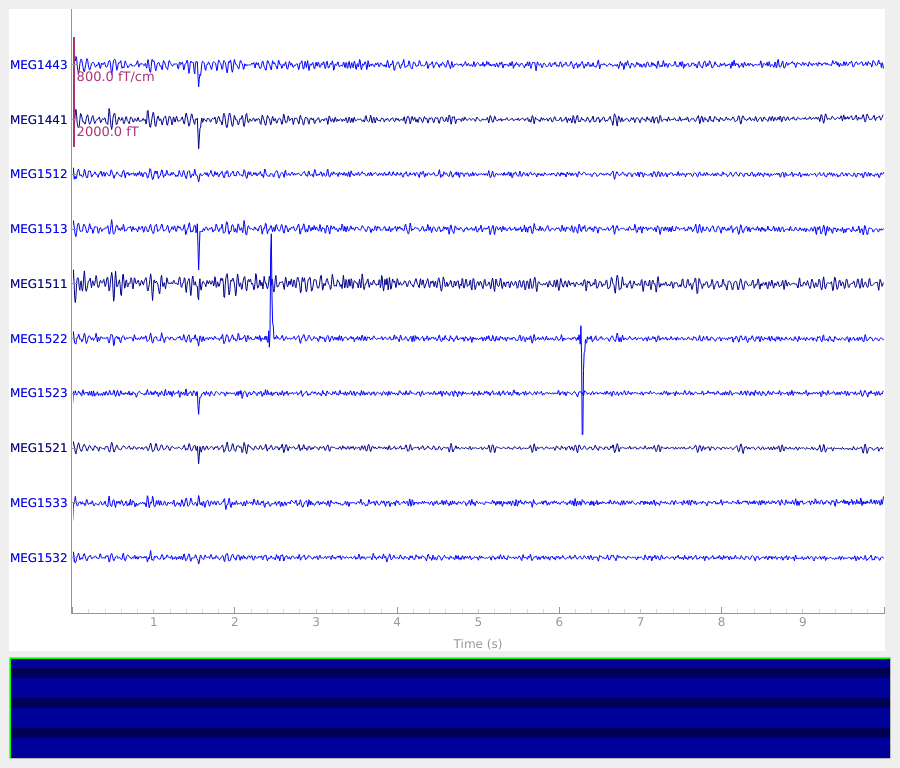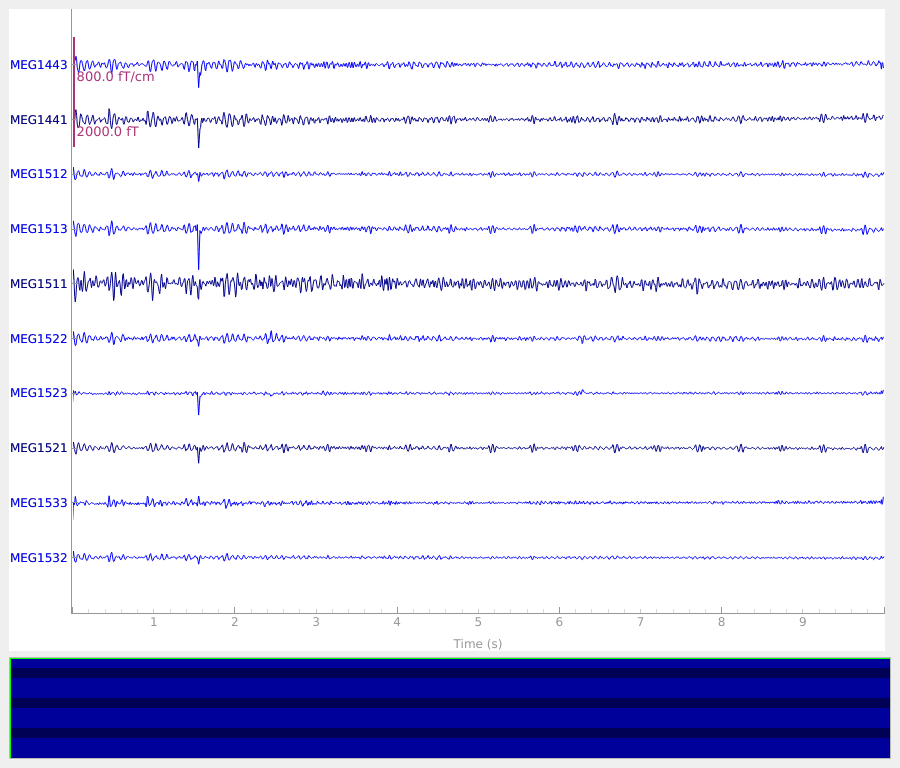# Plot sensor denoising using oversampled temporal projection#

This demonstrates denoising using the OTP algorithm  on data with with sensor artifacts (flux jumps) and random noise.

```# Author: Eric Larson <larson.eric.d@gmail.com>
#
```
```import numpy as np

import mne
from mne import find_events, fit_dipole
from mne.datasets.brainstorm import bst_phantom_elekta

print(__doc__)
```

Plot the phantom data, lowpassed to get rid of high-frequency artifacts. We also crop to a single 10-second segment for speed. Notice that there are two large flux jumps on channel 1522 that could spread to other channels when performing subsequent spatial operations (e.g., Maxwell filtering, SSP, or ICA).

```dipole_number = 1
data_path = bst_phantom_elekta.data_path()
order = list(range(160, 170))
raw.copy().filter(0.0, 40.0).plot(order=order, n_channels=10)
``````Opening raw data file /home/circleci/mne_data/MNE-brainstorm-data/bst_phantom_elekta/kojak_all_200nAm_pp_no_chpi_no_ms_raw.fif...
Read a total of 13 projection items:
planar-0.0-115.0-PCA-01 (1 x 306)  idle
planar-0.0-115.0-PCA-02 (1 x 306)  idle
planar-0.0-115.0-PCA-03 (1 x 306)  idle
planar-0.0-115.0-PCA-04 (1 x 306)  idle
planar-0.0-115.0-PCA-05 (1 x 306)  idle
axial-0.0-115.0-PCA-01 (1 x 306)  idle
axial-0.0-115.0-PCA-02 (1 x 306)  idle
axial-0.0-115.0-PCA-03 (1 x 306)  idle
axial-0.0-115.0-PCA-04 (1 x 306)  idle
axial-0.0-115.0-PCA-05 (1 x 306)  idle
axial-0.0-115.0-PCA-06 (1 x 306)  idle
axial-0.0-115.0-PCA-07 (1 x 306)  idle
axial-0.0-115.0-PCA-08 (1 x 306)  idle
Range : 47000 ... 437999 =     47.000 ...   437.999 secs
Reading 0 ... 10000  =      0.000 ...    10.000 secs...
Filtering raw data in 1 contiguous segment
Setting up low-pass filter at 40 Hz

FIR filter parameters
---------------------
Designing a one-pass, zero-phase, non-causal lowpass filter:
- Windowed time-domain design (firwin) method
- Hamming window with 0.0194 passband ripple and 53 dB stopband attenuation
- Upper passband edge: 40.00 Hz
- Upper transition bandwidth: 10.00 Hz (-6 dB cutoff frequency: 45.00 Hz)
- Filter length: 331 samples (0.331 s)

[Parallel(n_jobs=1)]: Done  17 tasks      | elapsed:    0.0s
[Parallel(n_jobs=1)]: Done  71 tasks      | elapsed:    0.0s
[Parallel(n_jobs=1)]: Done 161 tasks      | elapsed:    0.1s
[Parallel(n_jobs=1)]: Done 287 tasks      | elapsed:    0.1s
```

Now we can clean the data with OTP, lowpass, and plot. The flux jumps have been suppressed alongside the random sensor noise.

```raw_clean = mne.preprocessing.oversampled_temporal_projection(raw)
raw_clean.filter(0.0, 40.0)
raw_clean.plot(order=order, n_channels=10)
``````Processing MEG data using oversampled temporal projection
Processing    1 data chunk of (at least) 10.0 s with 5.0 s overlap and hann windowing
The final 0.001 s will be lumped into the final window
Denoising     0.00 –    10.00 s
Denoising    10.00 –    10.00 s
Filtering raw data in 1 contiguous segment
Setting up low-pass filter at 40 Hz

FIR filter parameters
---------------------
Designing a one-pass, zero-phase, non-causal lowpass filter:
- Windowed time-domain design (firwin) method
- Hamming window with 0.0194 passband ripple and 53 dB stopband attenuation
- Upper passband edge: 40.00 Hz
- Upper transition bandwidth: 10.00 Hz (-6 dB cutoff frequency: 45.00 Hz)
- Filter length: 331 samples (0.331 s)

[Parallel(n_jobs=1)]: Done  17 tasks      | elapsed:    0.0s
[Parallel(n_jobs=1)]: Done  71 tasks      | elapsed:    0.0s
[Parallel(n_jobs=1)]: Done 161 tasks      | elapsed:    0.1s
[Parallel(n_jobs=1)]: Done 287 tasks      | elapsed:    0.1s
```

We can also look at the effect on single-trial phantom localization. See the Brainstorm Elekta phantom dataset tutorial for more information. Here we use a version that does single-trial localization across the 17 trials are in our 10-second window:

```def compute_bias(raw):
events = find_events(raw, "STI201", verbose=False)
events = events[1:]  # first one has an artifact
tmin, tmax = -0.2, 0.1
epochs = mne.Epochs(
raw,
events,
dipole_number,
tmin,
tmax,
baseline=(None, -0.01),
verbose=False,
)
cov = mne.compute_covariance(epochs, tmax=0, method="oas", rank=None, verbose=False)
idx = epochs.time_as_index(0.036)
data = epochs.get_data(copy=False)[:, :, idx].T
evoked = mne.EvokedArray(data, epochs.info, tmin=0.0)
dip = fit_dipole(evoked, cov, sphere, n_jobs=None, verbose=False)
actual_pos = mne.dipole.get_phantom_dipoles()[dipole_number - 1]
misses = 1000 * np.linalg.norm(dip.pos - actual_pos, axis=-1)
return misses

bias = compute_bias(raw)
print("Raw bias: %0.1fmm (worst: %0.1fmm)" % (np.mean(bias), np.max(bias)))
bias_clean = compute_bias(raw_clean)
print(
"OTP bias: %0.1fmm (worst: %0.1fmm)"
% (
np.mean(bias_clean),
np.max(bias_clean),
)
)
```
```Raw bias: 2.5mm (worst: 5.2mm)
OTP bias: 1.2mm (worst: 1.4mm)
```